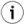### 9.19.5.Ordering and Grouping

The result rows of a query can be ordered based on their column values. The ORDER BY phrase allows specifying an ascending or descending sort order for a any column. The SQL interpreter will use an index if there is an index whose order reflects the order in the ORDER BY clause. If there is no appropriate index or if ascending and descending order is combined for columns of the same table the SQL interpreter will first evaluate the query and then sort the results before returning them.

See:Optimizations below for more information.
``` select * from Employees order by BirthDate;
```

will list all employees, oldest first, in ascending order of birth date.

The GROUP BY clause allows computing functions over repeating groups. Without the GROUP by clause set functions (AVG, MIN, MAX, SUM, COUNT) may not be mixed with normal columns in a selection list. If set functions and columns are mixed, all the columns must appear in the GROUP BY section. Such a query will produce as many rows as there are distinct value combinations of the grouping columns. The set functions will be computed for each distinct column combination.

```select OrderID, sum (UnitPrice * Quantity)
from Order_Details group by OrderID
having sum (UnitPrice * Quantity) > 5000  order by 2 desc;
```

Produces the OrderID and total value of the order in decreasing order of order value. The HAVING clause specifies that only orders with a value > 5000 will be counted. Note that the sum expression in having must be written identically to the same expression in the SELECT left.

The 2 in the order by refers to the second column of the select, which has no name, it being a function reference.

#### CUBE and ROLLUP

Virtuoso database offers a way to increase efficiency of SQL summary queries and simplify the operations. By using the options `ROLLUP` and `CUBE` in `GROUP BY` , can be executed more comprehensive summary operations. The produced result sets could be also produced without using these option but rahter with additional coding and queries.

The options `ROLLUP` and `CUBE` extend the result set of `GROUP BY` :

• `ROLLUP` : builds a consequence of subtotal aggregates on every queried level including the grand total.

• `CUBE` : this option is an extension of

`ROLLUP` . It builds all possible subtotal aggregates combinations for given

`GROUP BY` .

For example:

```  SELECT j, grouping (j), k, grouping (k), t, grouping (t), SUM (i)
FROM MyDemo
GROUP BY ROLLUP (j,k,t);

SELECT j, grouping (j), k, grouping (k), t, grouping (t), SUM (i)
FROM MyDemoCube
GROUP BY CUBE (j,k,t);
```

The `grouping(param)` procedure returns "1" if the column "param" is not in the dynamic GROUP BY set, and returns "0" otherwise.

The result set of:

```  SELECT j, k, t, SUM (i)
FROM MyDemo
GROUP BY ROLLUP (j,k,t) ;
```

is equivalent of the accumulated result sets of:

```  SELECT j, k, t, SUM (i)
FROM MyDemo
GROUP BY j,k,t;

SELECT NULL, k, t, SUM (i)
FROM MyDemo
GROUP BY k,t;

SELECT NULL, NULL, t, SUM (i)
FROM MyDemo
GROUP BY t;

SELECT NULL, NULL, NULL, SUM (i)
FROM MyDemo;
```

The result set of:

```SELECT t,s, SUM (i) FROM MyDemo GROUP BY CUBE (t,s);
```

is equivalent of the accumulated result set of:

```  SELECT t,s, SUM (i)
FROM MyDemo
GROUP BY t,s;

SELECT t,NULL, SUM (i)
FROM MyDemo
GROUP BY t;

SELECT s,NULL, SUM (i)
FROM MyDemo
GROUP BY s;

SELECT NULL,NULL, SUM (i)
FROM MyDemo;
```